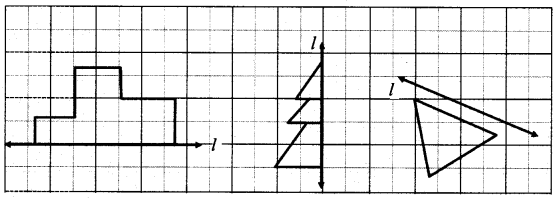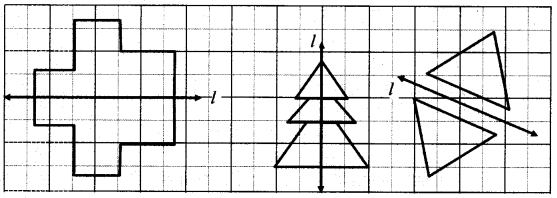# Maharashtra Board Practice Set 21 Class 6 Maths Solutions Chapter 7 Symmetry

Balbharti Maharashtra State Board Class 6 Maths Solutions covers the Std 6 Maths Chapter 7 Symmetry Class 6 Practice Set 21 Answers Solutions.

## 6th Standard Maths Practice Set 21 Answers Chapter 7 Symmetry

Question 1.
Along each figure shown below, a line l has been drawn. Complete the symmetrical figures by drawing a figure on the other side such that the line l becomes the line of symmetry.Solution:#### Maharashtra Board Class 6 Maths Chapter 7 Symmetry Practice Set 21 Intext Questions and Activities

Question 1.
In the figures below, the line l divides the figure in two parts. Do these parts fall on each other? Verify? (Textbook pg. no. 42)Solution:
Yes, the two parts of both the figures fall on each other on folding along the line l.